# Functions and the Writing of Code

• 53k Accesses

Part of the Texts in Computational Science and Engineering book series (TCSE,volume 15)

## Abstract

Until now, we have benefitted from using functions like, e.g., zeros and linspace from numpy. These have all been written by others for us to use. Now we will look at how to write such functions ourselves, an absolutely fundamental skill in programming.

## 4.1 Functions: How to Write Them?

Until now, we have benefitted from using functions like, e.g., zeros and linspace from numpy. These have all been written by others for us to use. Now we will look at how to write such functions ourselves, an absolutely fundamental skill in programming.

In its simplest form, a function in a program is much like a mathematical function: some input number x is transformed to some output number. One example is the $$\tanh ^{-1}(x)$$ function, called atan in computer code: it takes one real number as input and returns another number. Functions in Python are more general and can take a series of values as input and return one or more results, or simply nothing. The purpose of functions is twofold:

1. 1.

to group code lines that naturally belong together (making such units of code is a strategy which may ease the problem solving process dramatically), and

2. 2.

to parameterize a set of code lines such that they can be written only once and easily be re-executed with variations.

Functions that we write ourselves are often referred to as user-defined functions. Throughout this book, we will present many examples, in various contexts, of how such functions may be written.

### 4.1.1 Example: Writing Our First Function

To grasp the first few essentials about function writing, we change ball.py from Sect. 1.2, so that the height y is rather computed by use of a function that we define ourselves. Also, to better demonstrate the use of our function, we compute y at two points in time (not only one, as in ball.py). The new program ball_function.py then appears as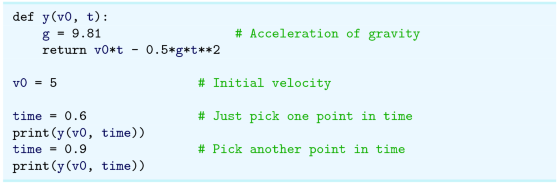### The Function Definition

When Python reads this program from the top, it takes the code from the line with def, to the line with return, to be the definition of a function by the name y. Note that this function (or any function for that matter) is not executed until it is called. Here, that happens with the two calls (y( v0, time) ) appearing in the print commands further down in the code. In other words, when Python reads the function definition for the first time, it just “stores the definition for later use” (and checks for syntax errors, see Exercise 4.8).

### Calling the Function

When the function is called the first time, the values of v0 (5) and time (0.6) are transferred to the y function such that, in the function, v0 = 5 and t = 0.6. Thereafter, Python executes the function line by line. In the final line return v0*t - 0.5*g*t**2, the expression v0*t - 0.5*g*t**2 is computed, resulting in a number which is “returned” to replace y( v0, time) in the calling code. The function print is then called with this number as input argument and proceeds to print it (i.e., the height). Alternatively, the number returned from y could have been assigned to a variable, e.g., like h = y( v0, time) , before printing as print( h) .

Python then proceeds with the next line, setting time to 0.9, before a new function call is triggered in the following line, causing a second execution of the function y and printout of the new height.

### Variable Names in Function Calls

Observe that, when calling the function y, the time was contained in the variable time, whereas the corresponding input variable (called a parameter) in the function y had the name t. We should reveal already now, that in general, variable names in function calls do not have to be the same as the corresponding names in the function definition. However, with v0 here, we see that variable names can be the same in the function call and the function definition (but technically, they are then two different variables with the same name, see Sect. 4.1.4). More rules about this will follow soon.

### Function Structure

We may now write up a more general form of a Python function as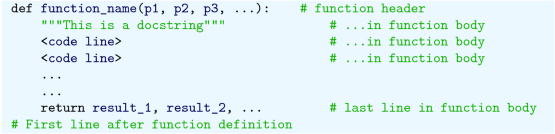A function definition must appear before the function is called, and usually, function definitions are placed one after the other at the top of a program, after import statements (if any).

### Function Header (Positional Parameters)

The first line, often called the function header, always starts with the reserved word def. This word is succeeded by the name of the function, followed by a listing of comma-separated names in parentheses, being the parameters of the function (here symbolized with p1, p2, p3,…). These parameters specify what input the function needs for execution. The header always ends with a colon. Function and parameter names must be decided by the programmer.

The function header may contain any numberFootnote 1 of parameters, also none. When there are no parameters, the parentheses must still be there, i.e., like def function_name():.

When input parameters are listed on the form above, they are referred to as positional parameters. Below, in Sect. 4.1.5, we will see what freedom there is when calling functions defined with such parameters.

### Another Typical Function Header (Keyword Parameters)

Another kind of function header that you often will deal with, is one which allows default values to be given to some, or all, of the input parameters. We may write such a header on the following formFootnote 2 (it could replace the previous header that has only positional parameters):In this case, the two first parameters are positional, whereas p3 and p4 are known as keyword parameters. This means that, unless other values are specified for p3 and p4 when calling the function, they will get the default values default_3 and default_4, respectively. This will soon (Sect. 4.1.7) be demonstrated and discussed in more detail through examples.

A function header may have only positional parameters, only keyword parameters, or some of each. However, positional parameters must always be listed before keyword parameters (as shown in the header above).

Note that there should be no space to each side of = when specifying keyword parameters.

### Function Body

All code lines inside a function must be indented, conventionally with 4 spaces, or more (if there are more indentation levels). In a function, these indented lines represent a block of statements, collectively referred to as the function body. Once the indent is reversed, we are outside (and after) the function. Here, the comment # First line after function definition is after the function.

The first line in the function body shown here, is an optional documentation string, a docstring. This string is meant for a human interpreter, so it should say something about the purpose with the function and explain input parameters and return values, unless obvious. By convention, a docstring is enclosed in triple double quotes ("""), which allows the string to run over several lines. When present, the docstring must appear immediately after the function header, as shown above.

The code lines of a function are executed only when the function is called (or invoked), as explained above with ball_function.py. Usually, a function ends with a return statement, starting with the reserved word return, which “returns” one or more results back to the place where the function was called. However, the explicit return statement may be dropped if not needed.Footnote 3 How to receive multiple return values from a function call will be exemplified in Sect. 4.1.6.

A function body can have loops, branching and calls to other functions, and may contain as many code lines as you need. Sometimes, a function body contains nothing more than a return statement of the form return <some calculation>, where some calculation is carried out before returning the result. Note that function input parameters are not required to be numbers. Any object will do, e.g., strings or other functions.

### 4.1.3 Functions and the Main Program

An expression you will often encounter in programming, is main program, or that some code is “in main”. This is nothing particular to Python, and simply refers to that part of the program which is outside functions, taking function headers as part of the main program.

We may exemplify this with ball_function.py from above, using comments to tell which lines are not “in main”.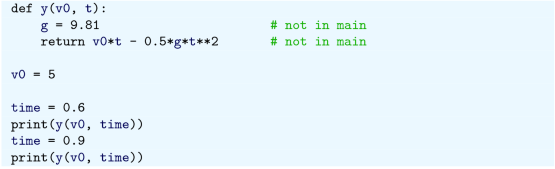Thus, everything is in main except the two lines of the function body.

### 4.1.4 Local Versus Global Variables

In our program ball_function.py, we have defined the variable g inside the function y. This makes g a local variable, meaning that it is only known inside the function. Thus, if we had tried to use g outside of the function, we would have got an error message. To see this, we may insert print( v0*t - 0.5*g*t**2) as a new last line in main (i.e., after the last print( y( v0, time) ) ) and try to run the code. Now, Python will not recognize g, even if Python just used it inside the function y (you should try this to see for yourself, but remember to remove the inserted code after the test!).

The variables v0 and time are defined outside the function and are therefore global variables. They are known both outside and inside the function.Footnote 4

Input parameters listed in a function header are by rule local variables inside the function. If you define one global and one local variable, both with the same name (as v0 in ball_function.py), the function body only “sees” the local one, so the global variable is not affected by what happens to its local “name-brother”.

If you want to change the value of a global variable inside a function, you need to declare the variable as global inside the function. That is, if some global variable was named x, we would need to write global x inside the function definition before we let the function change it. After function execution, x would then have a changed value.

### 4.1.5 Calling a Function Defined with Positional Parameters

We will here discuss alternative ways of calling the function y from ballnrea_function.py. This function has only got positional parameters, and throughout, the definitionis kept unchanged.

### Parameter Versus Argument

As explained previously, the input variables specified in a function definition are called the parameters of the function. When we call that function, however, the values provided in the call are referred to as arguments.Footnote 5

### Mixing the Position of Arguments

Assume now, like before, that we just want our main program to compute the height of the ball after 0.6 s, when the initial velocity is 5 ms −1. In our main program, we could then try two alternative calls,Here, y is called with positional arguments only (also termed ordinary arguments). The first alternative will do the job. Regarding the second alternative, it will print a result, but a wrong one, since arguments are in the wrong position when calling. With the second call to y (i.e., y( 0.6, 5) ), we get v0 = 0.6 and t = 5 in the function, which clearly is not what we intended. Note that Python has no way of knowing that this is wrong, so it will happily compute a height according to the function definition and print an answer.

Doing the same with variables, e.g., likewill of course not change anything. The first call will give the correct result, while the latter will print the wrong result (without any error message).

### Using Keywords in the Call

It is possible to use function input parameter names as keywords when calling the function (note, the function definition is still unchanged with only positional parameters!). This brings the advantage of making the function call more readable. Also, it allows the order of arguments to be switched, according to some rules. A few examples will illustrate how this works.

By use of the parameter names v0 and t from the function definition, we may have the following statements in main: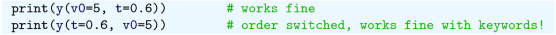Here, y is called with keyword arguments only (also termed named arguments). Either of the two alternative calls will give the correct printout, since, irrespective of argument ordering, Python is explicitly told which function parameter should have which value. This generalizes: as long as keywords are used for all arguments in a function call, any ordering of arguments can be used.

It is allowed to have a mix of positional and keyword arguments in the call, however, but then we need to be a bit more careful. With the following lines in main,the first alternative is acceptable, while the second is not. The general rule is, that when mixing positional and keyword arguments, all the positional arguments must precede the keyword arguments. The positional arguments must also come in the very same order as the corresponding input parameters appear in the function definition, while the order of the keyword arguments may be changed.

Note that, if some function is defined with a “long” list of input parameters, when calling that function, you can not use a keyword for one of the arguments “in the middle”, even if placed last in the list (make yourself an example with 3 parameters and test it!). This is because Python reads the listed arguments from left to right, and does not know that an argument is taken out of the list before it comes to the end (but then it is too late).

### 4.1.6 A Function with Two Return Values

Take a slight variation of our case with the ball, assuming that the ball is not thrown straight up, but at an angle, so that two coordinates are needed to specify its position at any time.

According to Newton’s laws (when air resistance is negligible), the vertical position is given by y(t) = v 0y t − 0.5gt 2 and, simultaneously, the horizontal position by x(t) = v 0x t. We can include both these expressions in a new version of our program that finds the position of the ball at a given time:We note that in the return statement, the returned values (two here) are separated by a comma. Here, the x coordinate will be computed by v0x*t, while the y coordinate will be computed like before. These two calculations produce two numbers that are returned to variables x and y in main. Note that, the order of the results returned from the function must be the same as the order of the corresponding variables in the assignment statement. Note the use of a comma both in the return statement and the assignment statement.

### 4.1.7 Calling a Function Defined with Keyword Parameters

Let us adjust the previous program slightly, introducing keyword parameters in the definition. For example, if we use 0.6 as a default value for t, and aim to get the same printout as before, the program reads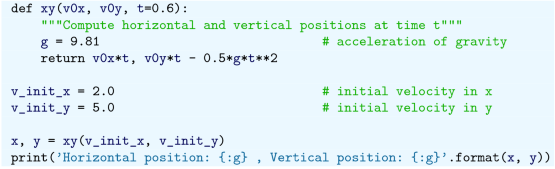Alternatively, with default values for all the parameters, and again aiming for the same printout, our program (ball_position_xy.py) appears asRunning the code, we get the printout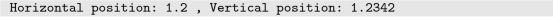Some, or all, of the default values may also be overridden, e.g., likewhich means that xy will run with default values for v0x and t, while v0y will have the value 6.0, as specified in the function call. The output then, becomes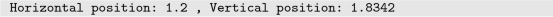which is reasonable, since the initial velocity for the vertical direction was higher than in the previous case.

### 4.1.8 A Function with Another Function as Input Argument

Functions are straightforwardly passed as arguments to other functions. This is illustrated by the following script function_as_argument.py, where a function sums up function values of another function: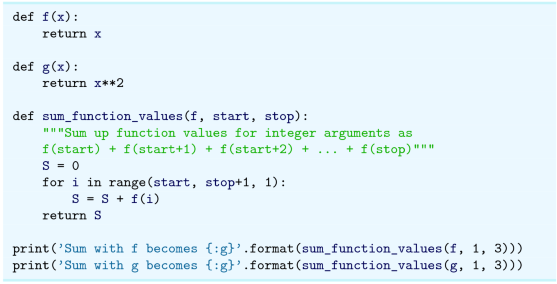We note that the function sum_function_values takes a function as its first argument and repeatedly calls that function without (of course) knowing what that function does, it just gets the function values back and sums them up!

Remember that the argument f in the function header sum_funcion_values (f, start, stop) and the function defined by the name f, is not the same. The function argument f in sum_funcion_values(f, start, stop) will act as the local “nick-name” (inside the function sum_funcion_values) for any function that is used as first argument when calling sum_funcion_values.

Executing the program, gives the expected printout as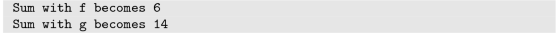### 4.1.9 Lambda Functions

A one-line function may be defined in a compact way, as a so-called lambda function. This may be illustrated as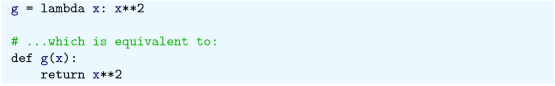Lambda functions are particularly convenient as function arguments, as seen next, when calling sum_function_values from above with lambda functions replacing the functions f and g: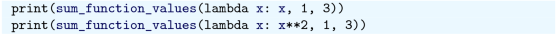This gives the same output as we got above. In this way, the lambda function is defined “in” the function call, so we avoid having to define the function separately prior to the (function) call.

A lambda function may take several comma-separated arguments. A more general form of a lambda function is thus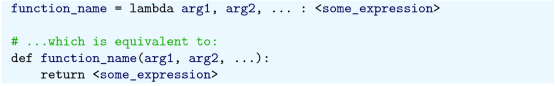The general syntax consists of the reserved word lambda, followed by a series of parameters, then a colon, and, finally, some Python expression resulting in an object to be returned from the function.

### 4.1.10 A Function with Several Return Statements

It is possible to have several return statements in a function, as illustrated here: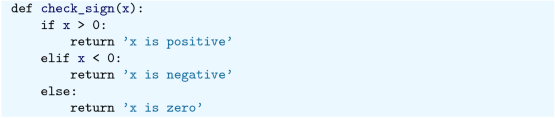Remember that only one of the branches is executed for a single call to check_sign, so depending on the number x, the return may take place from any of the three return alternatives.

### To Return at the End or Not

Programmers disagree whether it is a good idea to use return inside a function, or if there should be just a single return statement at the end of the function. The authors of this book emphasize readable code and think that return can be useful in branches as in the example above when the function is short. For longer or more complicated functions, it might be better to have one single return statement at the end.

### Nested Functions

Functions may also be defined within other functions. In that case, they become local functions, or nested functions, known only to the function inside which they are defined.

Functions defined in main are referred to as global functions. A nested function has full access to all variables in the parent function, i.e. the function within which it is defined.

### Overhead of Function Calls

Function calls have the downside of slowing down program execution. Usually, it is a good thing to split a program into functions, but in very computing intensive parts, e.g., inside long loops, one must balance the convenience of calling a function and the computational efficiency of avoiding function calls. It is a good rule to develop a program using plenty of functions and then in a later optimization stage, when everything computes correctly, remove function calls that are quantified to slow down the code. Let it be clear, however, that newcomers to programming should focus on writing readable code, not fast code!

In Sect. 5.6, we investigate (for a particular case) the impact that function calls have on CPU time.

## 4.2 Programming as a Step-Wise Strategy

When students start out with programming, they usually pick up the basic ideas quite fast and learn to read simpler code rather swiftly. However, when it comes to the writing of code, many find it hard to even get started.

In this chapter, we will use an example to present a typical code writing process in detail. Our focus will be on a step-wise approach that is so often required, unless the programmer is experienced and the programming task is “small”.

Often, such a step-wise approach starts out with a very simple version of the final program you have in mind. You test and modify that simple version until it runs like you want. Then you include some more code, test and modify that version until it works fine. Then you include more code, test and modify, and so on. Each of these steps then brings you closer to your final target program. In some cases, all the steps are clear in advance, but often, new insight develops along the way, making it necessary to modify the plan. The step-wise approach is good also in that it allows you to get started with a step or two (that you see are needed), even if you do not know how to proceed from there, at least yet.

How to break up a programming task into a series of steps is not unique. It will also depend on the problem, as well as on the programmer. More experienced programmers can save time by writing the final version of some program in one go (or at least with few steps). Beginners, however, may greatly benefit from a step-wise procedure with the sufficient number of steps. The following example should illustrate the idea.

### The Programming Task

Write a program that tests the user’s knowledge of the times tables from 1 to 10.

### Breaking up the Task

There are many possible ways to do such a times tables testing, but our reasoning goes as follows. In this test, there will be 10 different questions for the 1 times table, 10 different questions for the 2 times table, and so on, giving a 100 different questions in total. We decide to ask each of those questions one time only. There are quite many questions, so we also allow the user to quit with Ctrl-c (i.e., hold the Ctrl key down while typing c) before reaching the end.

To code this, the first idea that possibly comes to mind, is to use a double for loop on a form like: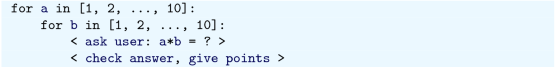With a construction like this, we see that for each value of a, the second factor b will run over all values 1 to 10. The questions will then appear in a predictable and systematic way. First we get the 1 times table (1*1, 1*2, …, 1*10), then the 2 times table (2*1, 2*2, …, 2*10), and so on. Clearly, this would be an acceptable approach. However, some would still argue that it might be better if the 100 questions were randomized, depriving the user any benefit from just remembering a sequence of answers.

Based on these reflections, we choose to break up the programming task into three steps:

• 1st version—has no dialogue with the user. It contains the double loop construction and two functions, ask_user and points. The function ask_user will (in later versions) ask the user for an answer to a*b, while points (in later versions) will check that answer, inform the user (correct or not), and give a score (1 point if correct). To simplify, the function bodies of these two functions will deliberately not be coded for this version of the program. Rather, we simply insert a print command in each, essentially printing the arguments provided in the call, to confirm that the function calls work as planned.

• 2nd version—asking and checking done systematically with predictable questions (first the 1 times table, then the 2 times table, etc.).

• 3rd version—asking and checking done with randomized questions. How to implement this randomization, will be kept as an open question till we get there.

(We do reveal, however, that something “unforeseen” will be experienced with the 3rd version, which will motivate us also for a 4th version of the program.)

### 4.2.2 The 1st Version of Our Code

Our very first version of the code (times_tables_1.py) may be written like this: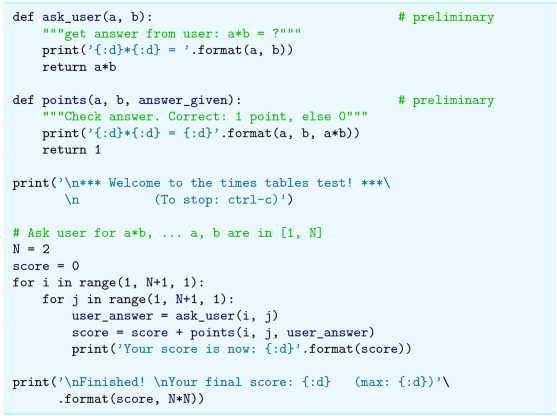In this implementation, the function ask_user will, by choice, not ask the user about anything. It will simply return the correct answer. Regarding points, it will return 1 without actually testing anything. In this way, we will be able to run the program, while still having unfinished parts in there.

We have introduced N as a variable here to allow easy adjustment of “problem size” (the total number of questions will be N*N). We know N must be 10, but that requirement applies to the final version only. Thus, we are free to do our steps with a smaller N, and that makes life much easier for us when assessing code behavior. If you have not already done so, go through the code by hand to confirm that you understand what happens, in what order.

When executed, the program simply prints: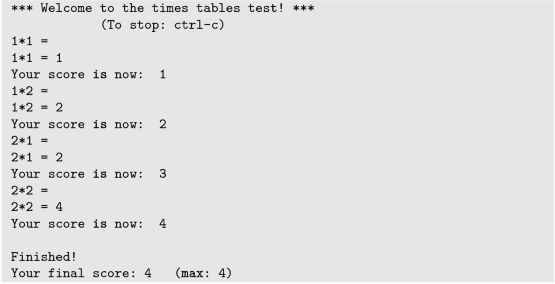From the printout, we see that the two functions seem to get the right arguments in each call. We are thus ready for the next step, i.e., to implement the function bodies of the two functions.

### 4.2.3 The 2nd Version of Our Code

After implementing the remaining parts of the code, we have a version (times_tables_2.py) of our program that actually does the testing that was asked for. The code reads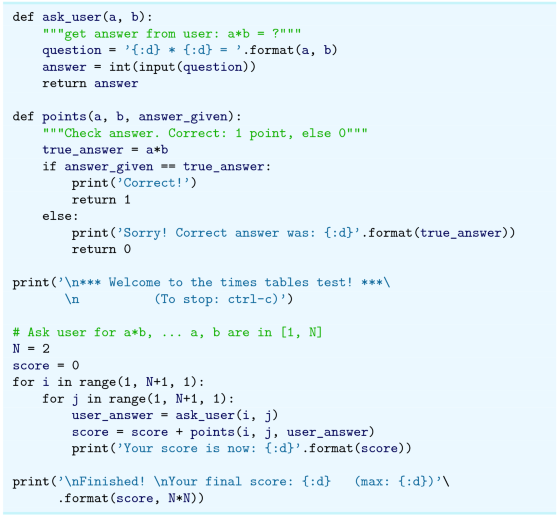Running the program, the dialogue could, for example, proceed like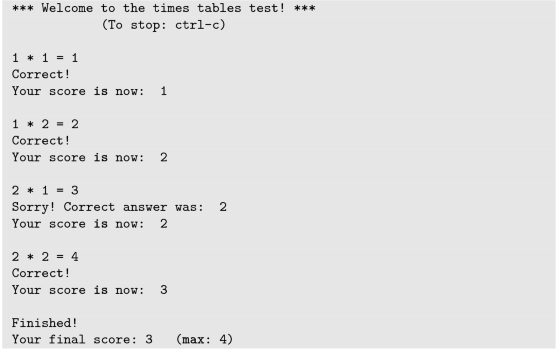We see that the behavior is as expected. Again, it is important that you read the code and confirm that your understanding is in line with the output shown.

### Testing for Equality with ==

In our code, we compare two integers in the if test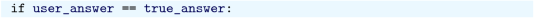Testing for equality with == works fine for the integers we have here. In general, however, such tests need to account for the inexact number representation that we have on computers. More about this in Sect. 6.6.3.

Note that the new code implemented when updating from times_tables_1.py to times_tables_2.py, included the function bodies in both functions ask_user and points. Often, however, it is a good idea to finalize one function at a time, particularly with larger and/or more complicated functions.

The reader should take a moment to reflect on the use of functions in general (ask_user and points here). They represent “logical units”, each dedicated to a special task. Structuring code this way, may greatly ease human code interpretation, which in turn makes debugging and future code changes much simpler. “Seen” from the main program, ask_user, for example, is given the factors of a*b and returns the answer. The inner workings of ask_user is neither known, nor of any concern, to the main program. It just calls the function and waits for the returned value. Moreover, the inner workings of ask_user may be changed in any way, without affecting the code elsewhere in the program, as long as function input and output stays the same.

By setting N = 10 in times_tables_2.py, and confirming that the dialogue runs correctly, we have a program that carries out the required test. However, we have yet another step to make, which means we have to figure out how the questions can be randomized. Let us see what we can make out of it.

### 4.2.4 The 3rd Version of Our Code

How can we randomize the 100 questions, while keeping to the plan of asking each question only once? Based on our previous version(s) of the code, it would be natural to first think of something like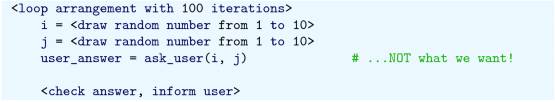However, it is immediately realized that some products a*b then will come several times, whereas others, are likely to not appear at all. Clearly, this is not what we want. Some more reasoning is required.

One solution, the one presented here, is based on two key observations. The first observation, is that the integers 0 to 99 can be used to uniquely represent each of our 100 products. We might demonstrate this by the following little piece of code:When executed, we get a printout likeThus, from the 100 values of i, we can uniquely derive the two factors in all the 100 products (!), as the printout confirms. With the sequence of i values just shown, however, we get the systematic ordering of the questions used in our 2nd version of the program. So, to get the questions in random order, we need something more.

The second observation, is that the function shuffle (Sect. 2.4) from numpy can be used to randomize the numbers 0 to 99, and thereby give us a randomized ordering of the products.

Now, based on these two observations, we are ready to write down the 3rd version of our program (times_tables_3.py), in which the functions ask_user and points are unchanged compared to the 2nd version: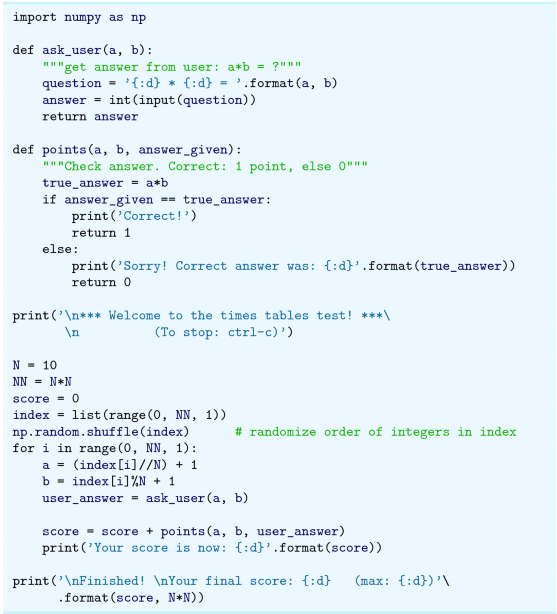Running this code, the order of the questions will be generated anew with each execution (because of the randomization), but the dialogue may, for example, appear like: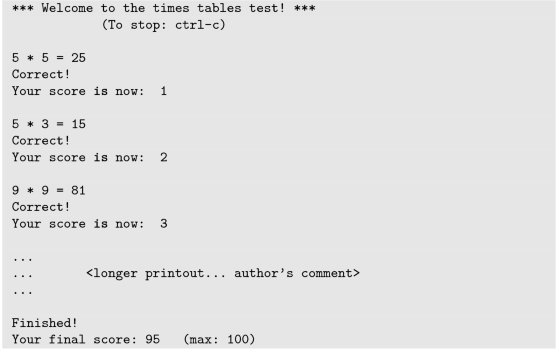Great! Our code seems to run smoothly, so what can possibly go wrong now?

This will go wrong: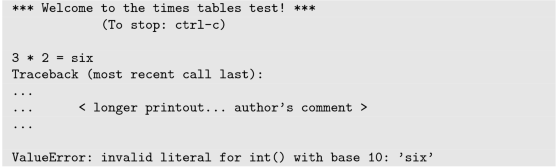If a user gives some unexpected input that the code is not prepared to handle, things can go very wrong! In this case, we get an error message (referring to some ValueError), since our program does not understand that “six” actually means the number 6.

It would not be very professional to leave our program with this potential problem, so it should be fixed, but how? The good news is that modern programming languages, Python inclusive, do have the right tools to deal with such cases. For now, we will leave our code as it is, but we hereby add yet another step to our program development plan, and will solve the problem when we turn to exception handling in Sect. 5.2. That will also bring us to the fourth version of our program, which also will be regarded as the final version (in general, however, programs are typically changed and improved again and again, making it hard to reach the “final” version!).

### Developing a Computational Plan

To write a program, you need to plan what that program should do, and a good plan requires a thorough understanding of the addressed problem. One fundamentally important thing with the step-wise strategy, is that it invites you to think through your computational problem very carefully: What bits and pieces, or “sub-problems”, make up the whole task? Should the “sub-problems” be solved in any particular order, i.e., do parts of the problem depend on results from other parts? What is the best way to compute each of the “sub-problems”?

This kind of thinking, which combines favorably with discussions among students/colleagues, often pays off in terms of a much deeper understanding of the problem at hand. Good solutions often require such an understanding.

### Compound Statements

The constructions met in this chapter, and the previous chapter, are characterized by a grouping of statements that generally span several lines (although it is possible to write simpler cases on a single line, when statements are separated with a semi-colon). Such constructions are often referred to as compound statements, having headers (ending with a colon) and bodies (with indented statements).Footnote 6

Interactive handling of compound statements is straight forward. For example, a for loop may be written (and executed) like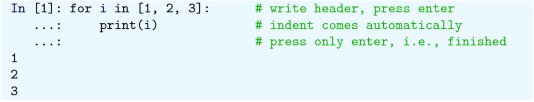When the header has been typed in and we press enter, we are automatically given the indent on the next line. We can then proceed directly by writing print( i) and press enter again. We then want to finish our loop, which is understood when we simply press enter, writing nothing else.

## 4.3 Exercises

### Exercise 4.1: Errors with Colon, Indent, etc.

Write the program ball_function.py as given in the text and confirm that the program runs correctly. Then save a copy of the program and use that program during the following error testing.

You are supposed to introduce errors in the code, one by one. For each error introduced, save and run the program, and comment how well Python’s response corresponds to the actual error. When you are finished with one error, re-set the program to correct behavior (and check that it works!) before moving on to the next error.

1. a)

Change the first line from def y( v0, t) : to def y( v0, t) , i.e., remove the colon.

2. b)

Remove the indent in front of the statement g = 9.81 inside the function y, i.e., shift the text four spaces to the left.

3. c)

Now let the statement g = 9.81 inside the function y have an indent of three spaces (while the remaining two lines of the function have four).

4. d)

Remove the left parenthesis in the first statement def y( v0, t) :

5. e)

Change the first line of the function definition from def y( v0, t) : to def y( v0) :, i.e., remove the parameter t (and the comma).

6. f)

Change the first occurrence of the command print( y( v0, time) ) to print( y( v0) ) .

Filename: errors_colon_indent_etc.py.

### Exercise 4.2: Reading Code 1

1. a)

Read the following code and predict the printout produced when the program is executed.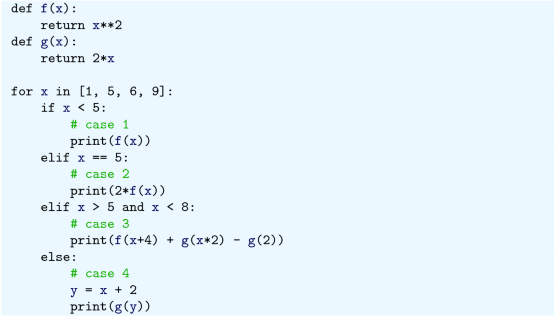1. b)

Type in the code and run the program to confirm that your predictions are correct.

### Exercise 4.3: Reading Code 2

1. a)

Read the following code and predict the printout produced when the program is executed.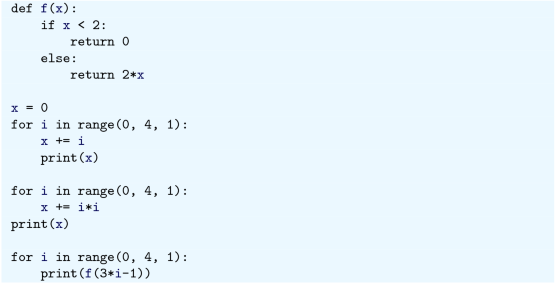1. b)

Type in the code and run the program to confirm that your predictions are correct.

### Exercise 4.4: Functions for Circumference and Area of a Circle

Write a program that takes a circle radius r as input from the user and then computes the circumference C and area A of the circle. Implement the computations of C and A as two separate functions that each takes r as input parameter. Print C and A to the screen along with an appropriate text. Run the program with r = 1 and confirm that you get the right answer.

Filename: functions_circumference_area.py.

### Exercise 4.5: Function for Adding Vectors

Write a function add_vectors that takes 2 vectors (arrays with one index are often called vectors) a and b as input arguments and returns the vector c, where c = a + b. Place the function in a little program that calls the function and prints the result. The function should check that a and b have the same length. If not, None should be returned. Confirm that your function works by comparing to hand calculations (i.e., just choose some arrays and test).

### Exercise 4.6: Function for Area of a Rectangle

Write a program that computes the area A = bc of a rectangle. The values of b and c should be user input to the program. Also, write the area computation as a function that takes b and c as input parameters and returns the computed area. Let the program print the result to screen along with an appropriate text. Run the program with b = 2 and c = 3 to confirm correct program behavior.

Filename: function_area_rectangle.py.

### Exercise 4.7: Average of Integers

Write a program that gets an integer N > 1 from the user and computes the average of all integers i = 1, …, N. The computation should be done in a function that takes N as input parameter. Print the result to the screen with an appropriate text. Run the program with N = 5 and confirm that you get the correct answer.

Filename: average_1_to_N.py.

### Exercise 4.8: When Does Python Check Function Syntax?

You are told that, when Python reads a function definition for the first time, it does not execute the function, but still checks the syntax.

Now, you come up with some code lines to confirm that this is the case.

Hint

You may, for example, use a print command and a deliberate syntax error in a modification of ball_function.py (note that the modified code is one of those quick “one-time” tests you might make for yourself, meant to be deleted once you have the answer).

Filename: when_check_function_syntax.py.

### Exercise 4.9: Find Crossing Points of Two Graphs

Consider two functions f(x) = x and g(x) = x 2 on the interval [−4, 4].

Write a program that, by trial and error, finds approximately for which values of x the two graphs cross, i.e., f(x) = g(x). Do this by considering N equally distributed points on the interval, at each point checking whether |f(x) − g(x)| < 𝜖, where 𝜖 is some small number. Let N and 𝜖 be user input to the program and let the result be printed to screen. Run your program with N = 400 and 𝜖 = 0.01. Explain the output from the program. Finally, try also other values of N, keeping the value of 𝜖 fixed. Explain your observations.

Filename: crossing_2_graphs.py.

### Exercise 4.10: Linear Interpolation

Some measurements y i, i = 0, 1, …, N, of a quantity y have been collected regularly, once every minute, at times t i = i, i = 0, 1, …, N. We want to find the value y in between the measurements, e.g., at t = 3.2 min. Computing such y values is called interpolation.

Let your program use linear interpolation to compute y between two consecutive measurements:

1. 1.

Find i such that t i ≤ t ≤ t i+1.

2. 2.

Find a mathematical expression for the straight line that goes through the points (i, y i) and (i + 1, y i+1).

3. 3.

Compute the y value by inserting the user’s time value in the expression for the straight line.

1. a)

Implement the linear interpolation technique in a function interpolate that takes as input an array with the y i measurements, the time between them Δt, and some time t, for which the interpolated value is requested. The function should return the interpolated y value at time t.

2. b)

Write another function find_y that finds and prints an interpolated y value at times requested by the user. Let find_y use a loop in which the user is asked for a time on the interval [0, N]. The loop can terminate when the user gives a negative time.

3. c)

Use the following measurements: 4.4, 2.0, 11.0, 21.5, 7.5, corresponding to times 0, 1, …, 4 (min), and compute interpolated values at t = 2.5 and t = 3.1 min. Perform separate hand calculations to check that the output from the program is correct.

Filename: linear_interpolation.py.

### Exercise 4.11: Test Straight Line Requirement

Assume the straight line function f(x) = 4x + 1. Write a script that tests the “point-slope” form for this line as follows. Within a chosen interval on the x-axis (for example, for x between 0 and 10), randomly pick 100 points on the line and check if the following requirement is fulfilled for each point: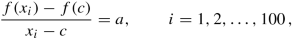where a is the slope of the line and c defines a fixed point (c, f(c)) on the line. Let c = 2 here.

Filename: test_straight_line.py.

### Exercise 4.12: Fit Straight Line to Data

Assume some measurements y i, i = 1, 2, …, 5 have been collected, once every second. Your task is to write a program that fits a straight line to those data.

1. a)

Make a function that, for given measurements and parameter values a and b, computes the error between the straight line f(x) = ax + b and the measurements:

\displaystyle \begin{aligned} e = \sum_{i=1}^{5} \left(ax_i+b - y_i\right)^2\, . \end{aligned}
2. b)

Make a function that, in a loop, asks the user to give a and b for the line. The corresponding value of e should then be computed and printed to screen, and a plot of the straight line f(x) = ax + b, together with the discrete measurements, should be produced.

3. c)

Given the measurements 0.5, 2.0, 1.0, 1.5, 7.5, at times 0, 1, 2, 3, 4, use the function in b) to interactively search for a and b such that e is minimized.

Filename: fit_straight_line.py.

Remarks

Fitting a straight line to measured data points is a very common task. The manual search procedure in c) can be automated by using a mathematical method called the method of least squares.

### Exercise 4.13: Fit Sines to Straight Line

A lot of technology, especially most types of digital audio devices for processing sound, is based on representing a signal of time as a sum of sine functions. Say the signal is some function f(t) on the interval [−π, π] (a more general interval [a, b] can easily be treated, but leads to slightly more complicated formulas). Instead of working with f(t) directly, we approximate f by the sum

\displaystyle \begin{aligned} S_N(t) = \sum_{n=1}^{N} b_n \sin{}(nt), \end{aligned}
(4.1)

where the coefficients b n must be adjusted such that S N(t) is a good approximation to f(t). We shall in this exercise adjust b n by a trial-and-error process.

1. a)

Make a function sinesum( t, b) that returns S N(t), given the coefficients b n in an array b and time coordinates in an array t. Note that if t is an array, the return value is also an array.

2. b)

Write a function test_sinesum() that calls sinesum( t, b) in a) and determines if the function computes a test case correctly. As test case, let t be an array with values − π∕2 and π∕4, choose N = 2, and b 1 = 4 and b 2 = −3. Compute S N(t) by hand to get reference values.

3. c)

Make a function plot_compare(f, N, M) that plots the original function f(t) together with the sum of sines S N(t), so that the quality of the approximation S N(t) can be examined visually. The argument f is a Python function implementing f(t), N is the number of terms in the sum S N(t), and M is the number of uniformly distributed t coordinates used to plot f and S N.

4. d)

Write a function error( b, f, M) that returns a mathematical measure of the error in S N(t) as an approximation to f(t):

\displaystyle \begin{aligned} E = \sqrt{\sum_{i} \left(f(t_i) - S_N(t_i)\right)^2},\end{aligned}

where the t i values are M uniformly distributed coordinates on [−π, π]. The array b holds the coefficients in S N and f is a Python function implementing the mathematical function f(t).

5. e)

Make a function trial( f, N) for interactively giving b n values and getting a plot on the screen where the resulting S N(t) is plotted together with f(t). The error in the approximation should also be computed as indicated in d). The argument f is a Python function for f(t) and N is the number of terms N in the sum S N(t). The trial function can run a loop where the user is asked for the b n values in each pass of the loop and the corresponding plot is shown. You must find a way to terminate the loop when the experiments are over. Use M=500 in the calls to plot_compare and error.

6. f)

Choose f(t) to be a straight line $$f(t) = \frac {1}{\pi }t$$ on [−π, π]. Call trial( f, 3) and try to find through experimentation some values b 1, b 2, and b 3 such that the sum of sines S N(t) is a good approximation to the straight line.

7. g)

Now we shall try to automate the procedure in f). Write a function that has three nested loops over values of b 1, b 2, and b 3. Let each loop cover the interval [−1, 1] in steps of 0.1. For each combination of b 1, b 2, and b 3, the error in the approximation S N should be computed. Use this to find, and print, the smallest error and the corresponding values of b 1, b 2, and b 3. Let the program also plot f and the approximation S N corresponding to the smallest error.

Filename: fit_sines.py.

Remarks

1. 1.

The function S N(x) is a special case of what is called a Fourier series. At the beginning of the nineteenth century, Joseph Fourier (1768–1830) showed that any function can be approximated analytically by a sum of cosines and sines. The approximation improves as the number of terms (N) is increased. Fourier series are very important throughout science and engineering today.

1. (a)

Finding the coefficients b n is solved much more accurately in Exercise 6.12, by a procedure that also requires much less human and computer work!

2. (b)

In real applications, f(t) is not known as a continuous function, but function values of f(t) are provided. For example, in digital sound applications, music in a CD-quality WAV file is a signal with 44,100 samples of the corresponding analog signal f(t) per second.

1. 1.

It is possible to have a variable number of input parameters (using *args and **kwargs). However, we do not pursue that any further here.

2. 2.

Strictly speaking, this header also incorporates the previous header, but they were split just to clarify the presentation.

3. 3.

In that case, something called None is returned, being a certain object that represents “nothing”.

4. 4.

The observant reader may then ask why not just define the function y without input parameters v0 and t, and simply use v0 and time directly inside the function (changing t to time in the return statement)? The answer is that this would have worked. However, there are several issues related to the use of global variables, so it is something that should be avoided in general.

5. 5.
6. 6.

Authors

## Rights and permissions

Open Access This chapter is licensed under the terms of the Creative Commons Attribution 4.0 International License (http://creativecommons.org/licenses/by/4.0/), which permits use, sharing, adaptation, distribution and reproduction in any medium or format, as long as you give appropriate credit to the original author(s) and the source, provide a link to the Creative Commons license and indicate if changes were made.

The images or other third party material in this chapter are included in the chapter's Creative Commons license, unless indicated otherwise in a credit line to the material. If material is not included in the chapter's Creative Commons license and your intended use is not permitted by statutory regulation or exceeds the permitted use, you will need to obtain permission directly from the copyright holder.

Reprints and Permissions